• 高斯过程
高斯过程

在概率论和统计学中，高斯过程是观测值出现在一个连续域（例如时间或空间）的统计模型。在高斯过程中，连续输入空间中每个点都是与一个正态分布的随机变量相关联。此外，这些随机变量的每个有限集合都有一个多元正态分布。

高斯过程被认为是一种机器学习算法，是以惰性学习方式，利用点与点之间同质性的度量作为核函数，以从输入的训练数据预测未知点的值。其预测结果不仅包含该点的值，而同时包含不确定性的资料——它的一维高斯分布。

如下公式所示，y,x$y,x$是已知的输入数据（包含多个数据），x∗$x_*$是新来的点，预测y∗$y_*$的值。
P(y∗|y,x,x∗)=P(y∗,y|x,x∗)P(y|x,x∗)=P(y∗,y|x,x∗)P(y|x)
P(y_*|y,x,x_*)=\frac{P(y_*,y|x,x_*)}{P(y|x,x_*)}=\frac{P(y_*,y|x,x_*)}{P(y|x)}

显然，核心就是建立P(y|x)$P(y|x)$的具体形式，然后求出条件概率P(y∗|y,x,x∗)$P(y_*|y,x,x_*)$。

高斯过程回归

现实生活中，我们遇到的一个典型问题就是选择合适的模型拟合训练集中自变量x$x$与因变量y$y$之间的关系，并根据新的自变量x∗$x_*$来预测对应的因变量y∗$y_*$
P(y∗|x,y,x∗)
P(y_*|x,y,x_*)

如果关系足够简单，那么线性回归就能实现很好的预测，但现实情况往往十分复杂，此时，高斯过程回归就为我们提供了拟合复杂关系的绝佳方法。模型的建立

普通线性回归：

y=wTx
y=w^Tx

线性无法满足，投影到高维（这里的wT$w^T$与上式的wT$w^T$维度不一样）：

y=wTϕ(x)
y=w^T\phi(x)

y$y$满足高斯过程（f(x)$f(x)$之间的协方差定义成与输入x$x$有关的函数，Δx$\Delta x$越小，相关性越高，协方差越大）：

y=wTϕ(x)+f(x)s.t. f(x)∼GP(0,k(x,x′))
y=w^T\phi(x)+f(x) \\
s.t. \space f(x)\sim GP(0, k(x,x'))

观测不准，带噪声：

y=wTϕ(x)+f(x)+ϵs.t. f(x)∼GP(0,k(x,x′)),ϵ∼N(0,σ2)
y=w^T\phi(x)+f(x)+\epsilon \\
s.t. \space f(x)\sim GP(0, k(x,x')), \\
\epsilon\sim N(0,\sigma^2)

写成向量形式，该模型等价于：

P(y|f,x)=N(y|Φw+f,σ2I),P(f|x)=N(f|0,K(x,x)).(y=[y1,y2,...,yn]T,x=[xT1,xT2,...,xTn]T,Φ=[ϕ(x1)T;ϕ(x2)T;...ϕ(xn)T])
P(y|f,x) = N(y|\Phi w +f, \sigma^2 I),\\
P(f|x) = N(f|0,K(x,x)). \\
(y = [y_1, y_2, ...,y_n]^T,\\
x = [x_1^T, x_2^T, ...,x_n^T]^T,\\
\Phi = [\phi(x_1)^T; \phi(x_2)^T; ...\phi(x_n)^T])

模型的求解

超参数估计（极大似然）

已有观测数据，要去估计概率分布的参数，最直接的想法就是使用极大似然估计了。把K(x,x)$K(x,x)$简写成K$K$，所有要估计的参数如下：
w,σ,θK
w,\sigma,\theta_K

两个独立高斯分布的和仍然是一个高斯分布：
ABA+B∼N(μ1,σ21)∼N(μ2,σ22)∼N(μ1+μ2,σ21+σ22)
\begin{align}
A &\sim N(\mu_1,\sigma_1^2)\\
B &\sim N(\mu_2,\sigma_2^2)\\
A+B &\sim N(\mu_1+\mu_2,\sigma_1^2+\sigma_2^2)\\
\end{align}

多维高斯分布：
N(x|μ,σ2)=1(2π)n/2|Σ|1/2exp(−12(x−μ)TΣ−1(x−μ))
N(x|\mu, \sigma^2)=\frac{1}{(2\pi)^{n/2}|\Sigma|^{1/2}}exp(-\frac{1}{2}(x-\mu)^T\Sigma^{-1}(x-\mu))

所以
yP(y|x)logP(y|x)=Φw+N(0,K)+N(0,σ2I)=P(y|x,w,σ,θK)=N(y|Φw,K+σ2I)=−n2log2π−12log|Σ|−12(y−Φw)TΣ−1(y−Φw)
\begin{align}
y&=\Phi w+N(0,K)+N(0,\sigma^2I)\\
P(y|x)&=P(y|x,w,\sigma,\theta_K) = N(y|\Phi w, K+\sigma^2I)\\
\log P(y|x)&=-\frac{n}{2} \log {2\pi} - \frac{1}{2} \log {|\Sigma|} - \frac{1}{2}(y-\Phi w)^T\Sigma^{-1}(y-\Phi w)\\
\end{align}

最大化以上函数求得最优参数：
w^=(ΦTΣ−1Φ)−1(ΦTΣ−1y)σ^,θK^=...
\hat {w} = (\Phi ^T\Sigma^{-1}\Phi)^{-1} (\Phi^T \Sigma^{-1}y) \\
\hat{\sigma}, \hat{\theta_K} = ...

预测

如我们开始所说，y,x$y,x$是已知的输入数据（包含多个数据），x∗$x_*$是新来的点，预测y∗$y_*$的值:
P(y∗|y,x∗,x)=P(y∗,y|x∗,x)P(y|x∗,x)=P(y∗,y|x∗,x)P(y|x)
P(y_*|y,x_*,x)=\frac{P(y_*,y|x_*,x)}{P(y|x_*,x)}=\frac{P(y_*,y|x_*,x)}{P(y|x)}

（思考：既然高斯过程回归类似于线性回归，为什么我们不把x∗$x_*$直接代入公式P(y∗|x∗)$P(y_*|x_*)$求得y∗$y_*$？提示：协方差矩阵和高斯过程定义）
P(y∗,y|x∗,x)=∫∫P(y∗,y,f∗,f|x∗,x)df∗df=∫∫P(y∗,y|f∗,f,x∗,x)P(f∗,f|x∗,x)df∗df=∫∫P(y∗|f∗,x∗)P(y|f,x)P(f∗|f,x∗,x)P(f|x)df∗df=∫∫P(y∗|f∗,x∗)P(f∗|f,x∗,x)P(f|y,x)P(y|x)df∗df
\begin{align}
P(y_*,y|x_*,x) &=\int \int P(y_*,y,f_*,f|x_*,x)df_*df\\
&=\int \int P(y_*,y|f_*,f,x_*,x)P(f_*,f|x_*,x)df_*df\\
&=\int \int P(y_*|f_*,x_*)P(y|f,x)P(f_*|f,x_*,x)P(f|x)df_*df\\
&= \int \int P(y_*|f_*,x_*)P(f_*|f,x_*,x)P(f|y,x)P(y|x) df_*df\\
\end{align}

把上式P(y|x)$P(y|x)$提取出来并化简，可得
P(y∗|y,x,x∗)=∫∫P(y∗|f∗,x∗)P(f∗|f,x∗,x)P(f|y,x)df∗df
P(y_*|y,x,x_*)=\int \int P(y_*|f_*,x_*)P(f_*|f,x_*,x)P(f|y,x)df_*df\\

已知：
P(y∗|f∗,x∗)P(f|y,x)P(f∗|f,x∗,x)=N(y∗|ϕ(x∗)Tw+f∗,σ2)=P(y|f,x)P(f|x)P(y|x)=N(f|K(y−Φw)K+σ2I,Kσ2K+σ2I)=P(f∗,f|x∗,x)P(f|x)=...
\begin{align}
P(y_*|f_*,x_*)&=N(y_*|\phi(x_*)^Tw+f_*, \sigma^2) \\
P(f|y,x) &= \frac{P(y|f,x)P(f|x)}{P(y|x)}=N(f|\frac{K(y-\Phi w)}{K+\sigma^2I}, \frac{K\sigma^2}{K+\sigma^2I}) \\
P(f_*|f,x_*,x)&=\frac{P(f_*,f|x_*,x)}{P(f|x)} =...\\
\end{align}

积分最后求得：
P(y∗|y,x∗,x)μΣ=N(y∗|μ,Σ)=wTϕ(x∗)+KT∗(K+σ2I)−1(y−Φw)=K∗∗−KT∗(K+σ2I)−1K∗+σ2
\begin{align}
P(y_*|y,x_*,x)&=N(y_*|\mu,\Sigma) \\
\mu &= w^T\phi(x_*)+K_*^T(K+\sigma^2I)^{-1}(y-\Phi w) \\
\Sigma &= K_{**}-K_*^T(K+\sigma^2I)^{-1}K^*  +\sigma^2\\
\end{align}

由上式可见，求出来y∗$y_*$服从一个高斯分布，而不是线性回归得出来的一个数值wTϕ(x∗)$w^T\phi (x_*)$。且
E[y∗]=wTϕ(x∗)+KT∗αwhere α=(K+σ2I)−1(y−Φw)
E[y_*]=w^T\phi(x_*)+K_*^T\alpha\\
where \space \alpha =(K+\sigma^2I)^{-1}(y-\Phi w) \\

由上式可以直观地看到，y∗​$y_*​$的期望等于当前数据的线性方程wTϕ(x∗)​$w^T\phi(x_*)​$加上训练数据x​$x​$与当前数据相关性的加权和。

高斯过程去噪

输入：x - 坐标

输出：y - 噪声图像像素值注意：高斯过程回归计算速度有待提升，对于比较大的图像最好每次输入一张patch做回归，上图就是100x100的图像块。
展开全文• 高斯过程高斯过程回归: https://zhuanlan.zhihu.com/p/100443773

高斯过程和高斯过程回归
高斯过程回归GPR
多元高斯分布（The Multivariate normal distribution）


展开全文• 高斯过程分类和高斯过程回归Gaussian processing (GP) is quite a useful technique that enables a non-parametric Bayesian approach to modeling. It has wide applicability in areas such as regression, ...
高斯过程分类和高斯过程回归Gaussian processing (GP) is quite a useful technique that enables a non-parametric Bayesian approach to modeling. It has wide applicability in areas such as regression, classification, optimization, etc. The goal of this article is to introduce the theoretical aspects of GP and provide a simple example in regression problems. 高斯处理(GP)是一项非常有用的技术，可以采用非参数贝叶斯方法进行建模。 它在回归，分类，优化等领域具有广泛的适用性。本文的目的是介绍GP的理论方面，并为回归问题提供一个简单的示例。
Multivariate Gaussian distribution 多元高斯分布
We first need to do a refresher on multivariate Gaussian distribution, which is what GP is based on. A multivariate Gaussian distribution can be fully defined by its mean vector and covariance matrix 我们首先需要对多元高斯分布进行复习，这是GP所基于的。 多元高斯分布可以通过其均值向量和协方差矩阵完全定义
There are two important properties of Gaussian distributions that make later GP calculations possible: marginalization and conditioning. 高斯分布的两个重要属性使后来的GP计算成为可能：边际化和条件化。
Marginalization 边际化
With a joint Gaussian distribution, this can be written as, 使用联合高斯分布，可以写成：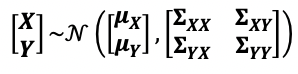We can retrieve a subset of the multivariate distribution via marginalization. For example, we can marginalize out the random variable Y, with the resulting X random variable expressed as follows, 我们可以通过边际化获取多元分布的子集。 例如，我们可以将随机变量Y边缘化，结果X随机变量表示如下，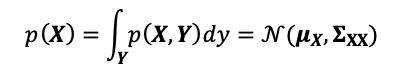Note that the marginalized distribution is also a Gaussian distribution. 注意，边缘分布也是高斯分布。
Conditioning 调理
Another important operation is conditioning, which describes the probability of a random variable given the presence of another random variable. This operation enables Bayesian inference, as we will show later, in deriving the predictions given the observed data. 另一个重要的操作是调节，它描述了在存在另一个随机变量的情况下一个随机变量的概率。 正如我们将在后面展示的那样，此操作启用贝叶斯推理，从而在给定观测数据的情况下得出预测。
With conditioning, you can derive for example, 通过调节，您可以得出例如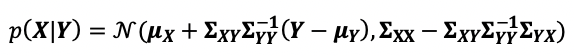Like the marginalization, the conditioned distribution is also a Gaussian distribution. This allows the results to be expressed in closed form and is tractable. 像边缘化一样，条件分布也是高斯分布。 这允许结果以封闭形式表示并且易于处理。
Gaussian process 高斯过程
We can draw parallels between a multivariate Gaussian distribution and a Gaussian process. A Gaussian process (GP) is fully defined by its mean function and covariance function (aka kernel), 我们可以在多元高斯分布和高斯过程之间得出相似之处。 高斯过程(GP)由其均值函数和协方差函数(即内核)完全定义，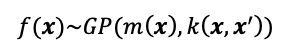GP can be thought of as an infinite dimensional multivariate Gaussian. This is actually what we mean by GP as being non-parametric — because there are an infinite number of parameters. The mean function, m(x), describes the mean of any given data point x, and the kernel, k(x,x’), describes the relationship between any given two data points x1 and x2. GP可以被视为无穷维多元高斯。 实际上，这就是我们所说的GP是非参数的-因为有无限数量的参数。 平均值函数m(x)描述任何给定数据点x的平均值，而内核k(x，x')描述任何给定的两个数据点x1和x2之间的关系。
As such, GP describes a distribution over possible functions. So when you sample from a GP, you get a single function. In contrast, when you sample from a Gaussian distribution, you get a single data point. 因此，GP描述了可能功能的分布。 因此，当您从GP采样时，您将获得一个功能。 相反，当从高斯分布中采样时，您将获得一个数据点。
Gaussian process regression 高斯过程回归
We can bring together the above concepts about marginalization and conditioning and GP to regression. In a traditional regression model, we infer a single function, Y=f(X). In Gaussian process regression (GPR), we place a Gaussian process over f(X). When we don’t have any training data and only define the kernel, we are effectively defining a prior distribution of f(X). We will use the notation f for f(X) below. Usually we assume a mean of zero, so all together this means, 我们可以将关于边际化和条件以及GP回归的上述概念组合在一起。 在传统的回归模型中，我们推导单个函数Y = f( X ) 。 在高斯过程回归(GPR)中，我们将高斯过程放在f( X )上。 当我们没有任何训练数据而仅定义内核时，我们实际上是在定义f( X )的先验分布。 我们将在下面的f ( X )中使用符号f 。 通常情况下，我们假设均值为零，所以所有这些都意味着，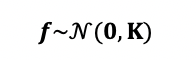The kernel K chosen (e.g. periodic, linear, radial basis function) describes the general shapes of the functions. The same way when you choose a first-order vs second-order equation, you’d expect different function shapes of e.g. a linear function vs a parabolic function. 所选的核K (例如，周期，线性，径向基函数)描述了函数的一般形状。 选择一阶方程与二阶方程时，采用相同的方式，您会期望不同的函数形状，例如线性函数与抛物线函数。
When we have observed data (e.g. training data, X) and data points where we want to estimate (e.g. test data, X*), we again place a Gaussian prior over f (for f(X)) and f* (for f(X*)), yielding a joint distribution, 当我们观察到数据(例如训练数据X )和要估计的数据点(例如测试数据X * )时，我们再次将高斯优先于f (对于f( X ) )和f * (对于f ( X * ) )，产生联合分布，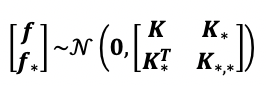The objective here is we want to know what is f* for some set of x values (X*) given we have observed data (X and its corresponding f). This is effectively conditioning, and in other words it is asking to derive the posterior probability of the function values, p(f*|f,X,X*). This is also how we can make predictions — to calculate the posterior conditioned on the observed data and test data points. 这里的目标是我们想知道给定的一组x值( X * )的f *是多少   我们已经观察到了数据( X及其对应的f )。 这是有效的条件，换句话说，它要求导出函数值p ( f * | f ， X ， X * )的后验概率。 这也是我们进行预测的方式-计算以观察到的数据和测试数据点为条件的后验。
The functions described above are noiseless, meaning we have perfect confidence in our observed data points. In the real world, this is not the case and we expect to have some noise in our observations. In the traditional regression models, this can be modeled as, 上述功能无噪音，这意味着我们对观察到的数据点具有完全的信心。 在现实世界中，情况并非如此，我们希望观察中会出现一些噪音。 在传统的回归模型中，可以将其建模为：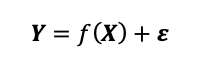where ε~N(0, σ² I). The ε is the noise term and follows a Gaussian distribution. In GPR, we place the Gaussian prior onto f(X) just like before, so f(X)~GP(0,K) and y(X)~GP(0, K+σ² I). With the observed data, the joint probability is very similar to before, except now with the added noise term to the observed data, 其中，ε〜N(0，σ²I)。 ε是噪声项，遵循高斯分布。 在GPR中，我们放置高斯先验到F(X)之前一样，因此f(X)〜GP(0，K)和y(X)〜GP(0，K +σ²I)。 对于观察到的数据，联合概率与以前非常相似，只是现在在观察到的数据中添加了噪声项，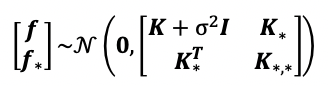Likewise, we can perform inference by calculating the posterior conditioned on f*, X, and X*. 同样，我们可以通过计算以f * ， X和X *为条件的后验条件来进行推断。
GPR using scikit-learn 使用scikit-learn进行GPR
There are multiple packages available for Gaussian process modeling (some are more general Bayesian modeling packages): GPy, GPflow, GPyTorch, PyStan, PyMC3, tensorflow probability, and scikit-learn. For simplicity, we will illustrate here an example using the scikit-learn package on a sample dataset. 有多种可用于高斯过程建模的软件包(有些是更通用的贝叶斯建模软件包)：GPy，GPflow，GPyTorch，PyStan，PyMC3，张量流概率和scikit-learn。 为了简单起见，我们将在示例数据集上使用scikit-learn包说明一个示例。
We will use the example Boston dataset from scikit-learn. First we will load and do a simple 80/20 split of the data into train and test sets. 我们将使用来自scikit-learn的示例波士顿数据集。 首先，我们将数据加载并进行简单的80/20拆分，将其分为训练集和测试集。
We will use the GaussianProcessRegressor package and define a kernel. Here we will try a radial-basis function kernel with noise and an offset. The hyperparameters for the kernel are suggested values and these will be optimized during fitting. 我们将使用GaussianProcessRegressor包并定义一个内核。 在这里，我们将尝试一个带有噪声和偏移量的径向基函数内核。 内核的超参数为建议值，这些参数将在拟合过程中进行优化。
You can view the fitted model with model.kernel_. We can now also plot and see our predicted versus actual, 您可以使用model.kernel_查看拟合的模型。 现在，我们还可以绘制并查看我们的预测值与实际值，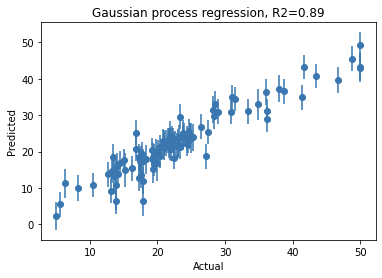Note that you can get similar performance with other machine learning models such as random forest regressor, etc. However, the key benefit from GPR is that for each given test data point, the predicted value naturally comes with confidence intervals. So not only do you know your model performance, but you know what is the uncertainty associated with each prediction. 请注意，您可以在其他机器学习模型(例如随机森林回归器等)上获得类似的性能。但是，GPR的主要好处是对于每个给定的测试数据点，预测值自然带有置信区间。 因此，您不仅知道模型的性能，而且知道与每个预测相关的不确定性是什么。
This is a high-level overview of GP and GPR. We won’t go into details of the kernels here. But by adopting different kernels, you can incorporate your prior assumptions about the data into your model. With the simple example with scikit-learn, we hope to provide some inspirations in seeing how GPR is useful and you can quickly get started to incorporate some form of Bayesian modeling as part of your machine learning toolbox! 这是GP和GPR的高级概述。 我们在这里不会详细介绍内核。 但是，通过采用不同的内核，您可以将有关数据的先前假设合并到模型中。 通过scikit-learn的简单示例，我们希望对GPR的有用性有所启发，并且您可以很快开始将某种形式的贝叶斯建模纳入机器学习工具箱！
Some other useful resources/posts about GP 有关GP的其他一些有用的资源/帖子
翻译自: https://towardsdatascience.com/getting-started-with-gaussian-process-regression-modeling-47e7982b534d高斯过程分类和高斯过程回归
展开全文python java 机器学习 人工智能
• Cambridge 高斯过程（Gaussian Process）讲义，高斯过程在空间-时序模型中具有重要作用，国内这块资料较少，与大家分享
• ## 高斯过程简析

千次阅读 多人点赞 2019-03-18 15:41:13
SLAM 方向对 Gaussian Process （GP）的需求不大，但这两年有好几篇 IROS，ICRA 的论文用高斯过程来拟合轨迹，拟合误差模型等，因此这篇笔记对高斯过程概念和原理进行简单梳理，理清楚 GP 是怎么来的，以及怎么用它...
前言
SLAM 方向对 Gaussian Process （GP）的需求不大，但这两年有好几篇 IROS，ICRA 的论文用高斯过程来拟合轨迹，拟合误差模型等，因此这篇笔记对高斯过程概念和原理进行简单梳理，理清楚 GP 是怎么来的，以及怎么用它。如果想更进一步系统学习下，推荐 MIT 出版的 Gaussian Processes for Machine Learning.
高斯过程是什么
高斯过程可以通俗的认为是函数拟合或回归近似，即给出变量 $\boldsymbol{x}$, 能预测出 $f(\boldsymbol{x})$。高斯分布是针对向量 $\boldsymbol{x}$ ，高斯过程则是对函数 $f(\boldsymbol{x})$。更学术一点的描述，高斯过程认为是一个服从均值是函数 $m(\boldsymbol{x})$，方差也是函数 $k\left(\boldsymbol{x}, \boldsymbol{x}^{\prime}\right)$ 的高斯分布 ，其中对任意变量 $\boldsymbol{x}, \boldsymbol{x}^{\prime}$ 有 $m(\boldsymbol{x})=\mathbb{E}[f(\boldsymbol{x})] \text { , } k\left(\boldsymbol{x}, \boldsymbol{x}^{\prime}\right)=\operatorname{Cov}\left(f(\boldsymbol{x}), f\left(x^{\prime}\right)\right)$。

举个简单的例子，一个函数 $f(\cdot)$ 的任意有限子集 $f\left(\boldsymbol{x}_{1}\right), \ldots, f\left(\boldsymbol{x}_{n}\right)$ 服从多元高斯分布，则称 $f(\cdot)$ 是一个高斯过程，其中输入 $\left\{\boldsymbol{x}_{n}\right\}_{n=1}^{N}$ 是任意维的向量。比如，$\left\{\boldsymbol{x}_{n}\right\}_{n=1}^{365}$ 表示 365 天，$f\left(\boldsymbol{x}_{n}\right)$ 表示某天的温度。

对于 N 组 D 维的输入数据 $\boldsymbol{X} \in \mathbb{R}^{N \times D}$，每一行表示一个输入（如24个小时时间点），协方差矩阵写为：
$\boldsymbol{K}_{\boldsymbol{X X}}=\left( \begin{array}{ccc}{k\left(\boldsymbol{x}_{1}, \boldsymbol{x}_{1}\right)} & {\cdots} & {k\left(\boldsymbol{x}_{1}, \boldsymbol{x}_{N}\right)} \\ {\vdots} & {\ddots} & {\vdots} \\ {k\left(\boldsymbol{x}_{N}, \boldsymbol{x}_{1}\right)} & {\cdots} & {k\left(\boldsymbol{x}_{N}, \boldsymbol{x}_{N}\right)}\end{array}\right)$
$k\left(\boldsymbol{x}, \boldsymbol{x}^{\prime}\right)$ 为核函数，通常只要求这个核函数是正定函数，好让 $\boldsymbol{K}_{\boldsymbol{X X}}$ 符合协方差矩阵的性质。比如对于服从零均值的 1 维变量 $\left\{x_{n}\right\}$ ，通常会用如下的核函数 (rational quadratic covariance function, RQ)：
$k\left(x, x^{\prime}\right)=h^{2}\left(1+\frac{\left(x-x^{\prime}\right)^{2}}{2 \alpha l^{2}}\right)^{-\alpha}$
其中 $h,\alpha, l$ 为正实数，我们称之为超参数，需要通过数据来训练得到。
**所以，给定一堆已有数据，怎么预测其他数据呢？**对于给定的输入 $\boldsymbol{X} \in \mathbb{R}^{N \times D}$ 以及对应的输出 $\boldsymbol{f} \in \mathbb{R}^{n}$, 当给定新的数据 $\boldsymbol{X}_{*}$ 时，我们要能预测出对应的 $\boldsymbol{f}_{*}$. 将训练集和测试集联合起来，得到多元高斯分布：
$\left( \begin{array}{c}{\boldsymbol{f}} \\ {\boldsymbol{f}_{*}}\end{array}\right) \sim \mathcal{N}\left(\left( \begin{array}{c}{\boldsymbol{m}_{\boldsymbol{X}}} \\ {\boldsymbol{m}_{\boldsymbol{X}_{*}}}\end{array}\right), \left( \begin{array}{c}{\boldsymbol{K}_{\boldsymbol{X X}} \quad \boldsymbol{K}_{\boldsymbol{X}\boldsymbol{X}_{*}}} \\ {\boldsymbol{K}_{\boldsymbol{X}_{*}\boldsymbol{X}} \quad \boldsymbol{K}_{\boldsymbol{X}_{*}\boldsymbol{X}_{*}}}\end{array}\right)\right)$
对于熟悉 SLAM 的同学来说，要从这个分布中计算出 $\boldsymbol{f}_{*}$, 采用舒尔补就行。从联合分布中计算  $\boldsymbol{f}_{*}$, 也就是算条件概率：
$p\left(\boldsymbol{f}_{*} | \boldsymbol{X}_{*}, \boldsymbol{X}, \boldsymbol{f}\right)=\mathcal{N}\left(\boldsymbol{m}_{\boldsymbol{X}_{*}}+\boldsymbol{K}_{\boldsymbol{X}_{*} \boldsymbol{X}} \boldsymbol{K}_{\boldsymbol{X} \boldsymbol{X}}^{-1}\left(\boldsymbol{f}-\boldsymbol{m}_{\boldsymbol{X}}\right), \boldsymbol{K}_{\boldsymbol{X}_{*} \boldsymbol{X}_{*}}-\boldsymbol{K}_{\boldsymbol{X}_{*} \boldsymbol{X}} \boldsymbol{K}_{\boldsymbol{X} \boldsymbol{X}}^{-1} \boldsymbol{K}_{\boldsymbol{X}\boldsymbol{X}_{*}}\right)$
对于不熟悉高斯分布这个操作的，可以参考这个回答。假设你已从训练数据中，得到了超参数，那就能用上述公司得到预测函数的分布，从而对于给定的 $\boldsymbol{X}_{*}$, 采样出  $\boldsymbol{f}_{*}$.
需要注意的是，训练的时候我们是用有噪声的数据 $y_{n}=f\left(\boldsymbol{x}_{n}\right)+\epsilon_{n}$ 来作为观测模型，其中 $\epsilon_{n} \sim \mathcal{N}\left(0, \sigma_{\epsilon}^{2}\right)$。而之前的核函数并没有假设这个噪声，因此可以将这个噪声加到协方差 $\boldsymbol{K}_{\boldsymbol{X X}}$ 的对角元素上。
高斯过程怎么训练
有了上述铺垫，训练其实就是最小二乘拟合那些超参数了。如有如下高斯过程，
\begin{aligned} f & \sim \mathcal{G P}(m, k) \\ m(x) &=a x^{2}+b x+c, \quad \text { and } \quad k\left(x, x^{\prime}\right)=\sigma_{y}^{2} \exp \left(-\frac{\left(x-x^{\prime}\right)^{2}}{2 \ell^{2}}\right)+\sigma_{\epsilon}^{2} \delta_{i i^{\prime}} \end{aligned}
其中，超参数为 $\theta=\left\{a, b, c, \sigma_{y}, \sigma_{n}, \ell\right\}$，对于训练集，可以最大化似然概率：
$L=\log p(\mathbf{y} | \mathbf{x}, \theta)=-\frac{1}{2} \log |K|-\frac{1}{2}(\mathbf{y}-\boldsymbol{m})^{\top} K^{-1}(\mathbf{y}-\boldsymbol{m})-\frac{n}{2} \log (2 \pi)$
然后使用高斯牛顿或者共而梯度法求解就行，只是其中涉及到求偏导数：
\begin{aligned} \frac{\partial L}{\partial \theta_{m}} &=-(\mathbf{y}-\boldsymbol{m})^{\top} \Sigma^{-1} \frac{\partial m}{\partial \theta_{m}} \\ \frac{\partial L}{\partial \theta_{k}} &=\frac{1}{2} \operatorname{trace}\left(K^{-1} \frac{\partial K}{\partial \theta_{k}}\right)+\frac{1}{2}(\mathbf{y}-\boldsymbol{m})^{\top} \frac{\partial K}{\partial \theta_{k}} K^{-1} \frac{\partial K}{\partial \theta_{k}}(\mathbf{y}-\boldsymbol{m}) \end{aligned}
通常采用数值求导或者自动微分就可以了。
结语
主要是 17 年, 1GA9 年 ICRA 有两篇关于高斯过程学习里程计误差模型的论文，以及 GTSAM 那边 Jing Dong 也有几篇把高斯过程用在 SLAM 里的，所以才有了兴趣大致梳理下这个知识。

2019 ICRA， Learning Wheel Odometry and Imu Errors for Localization.
2017 ICRA，Gaussian Process Estimation of Odometry Errors for Localization and Mapping

这篇博客主要参考了：

http://keyonvafa.com/gp-tutorial/
https://www.cs.ubc.ca/~hutter/EARG.shtml/earg/papers05/rasmussen_gps_in_ml.pdf


展开全文• 使用不同核函数的高斯过程 高斯过程 像所有其他机器学习模型一样，高斯过程是一个简单预测的数学模型。像神经网络一样，它可以用于连续问题和离散问题，但是其基础的一些假设使它不太实用。 但是，过去5年左右的时间...机器学习 深度学习 数据挖掘
• 高斯过程 高斯过程回归 高斯过程回归的应用步骤 1、选取合适的核函数，并计算核矩阵； 2、将核矩阵作为联合高斯分布的协方差矩阵，与历史数据联合计算条件概率分布； 3、由条件概率分布做线性回归预测。 ...
• 高斯过程是一种强大的回归和分类算法。它最大的优势是能够对自身的不确定性做出可靠的估计。本文目标是让您直观地了解什么是高斯过程。内容：机器学习如何应对不确定性贝叶斯推理高斯过程什么是机器学习？机器学习...
• matlab程序，高斯过程回归的实现，可以实现有效预测，根据本人之前的博客思路所写。有错误希望多多指正。回归预测
•机器学习算法
• 该程序是高斯过程经典程序 可用于分类 回归 预测等 Gaussian Processes for Machine Learning
• 高斯过程的理论知识非参数方法的基本思想高斯过程的基本概念高斯过程的Python实现使用Numpy手动实现使用Scikit-learn实现高斯过程小结高斯过程GaussianProcess高斯过程的理论知识非参数方法的基本思想在监督学习中...
• 这是高斯过程回归的代码，没用到gmpl工具箱的那种，希望能对大家有用！这是高斯过程回归的代码，没用到gmpl工具箱的那种，希望能对大家有用！
• ## 高斯过程 回归

热门讨论 2011-09-26 20:44:21
关于 高斯过程 的全套代码。。里面也有详细说明 有需要请拿去 关于 高斯过程 的全套代码。。里面也有详细说明 有需要请拿去
• 高斯过程模型是机器学习领域中的一个重要模型。本文我们首先给出形式化的问题定义和答案推导，接着按照答案给出对应的代码实现，验证这个答案的问题解决能力。最后我们再对形式化推导的过程进行一个比较详细的说明，...
• 代码里面是整个高斯过程类的实现，拟合了y=ax+b这样的直线。要运行的话，还要配上外围脚本，写点数据来驱动这个类。
• 高斯过程实现贝叶斯分类中描述的拉普拉斯近似，用于二元和多类分类。
• 很好用的高斯过程回归Matlab代码，可直接使用，欢迎大家下载
• 高斯过程回归是基于贝叶斯理论和统计学习理论发展起来的一种全新机器学习方法, 适于处理高维数、小 样本和非线性等复杂回归问题. 在阐述该方法原理的基础上, 分析了其存在的计算量大、噪声必须服从高斯分布等 问题......